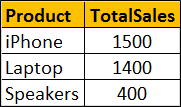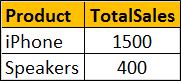HI WELCOME TO SIRING

# Difference between where and having in sql server

### we will discuss thedifference between where and havingclauses in SQL Server.

Let us understand the difference with an example. For the examples in this video we will use the following Sales table.SQL Script to create and populate Sales table with test data
Create table Sales
(
Product nvarchar(50),
SaleAmount int
)
Go

Insert into Sales values ('iPhone', 500)
Insert into Sales values ('Laptop', 800)
Insert into Sales values ('iPhone', 1000)
Insert into Sales values ('Speakers', 400)
Insert into Sales values ('Laptop', 600)
Go

To calculate total sales by product, we would write a GROUP BY query as shown below
SELECT Product, SUM(SaleAmount) AS TotalSales
FROM Sales
GROUP BY Product

The above query produces the following resultNow if we want to find only those products where the total sales amount is greater than \$1000, we will use HAVING clause to filter products
SELECT Product, SUM(SaleAmount) AS TotalSales
FROM Sales
GROUP BY Product
HAVING SUM(SaleAmount) > 1000

Result :If we use WHERE clause instead of HAVING clause, we will get a syntax error. This is because the WHERE clause doesn’t work with aggregate functions like sum, min, max, avg, etc.
SELECT Product, SUM(SaleAmount) AS TotalSales
FROM Sales
GROUP BY Product
WHERE SUM(SaleAmount) > 1000

So in short, the difference is WHERE clause cannot be used with aggregates where as HAVING can.

However, there are other differences as well that we need to keep in mind when using WHERE and HAVING clauses. WHERE clause filters rows before aggregate calculations are performed where as HAVING clause filters rows after aggregate calculations are performed. Let us understand this with an example.

Total sales of iPhone and Speakers can be calculated by using either WHERE or HAVING clause

Calculate Total sales of iPhone and Speakers using WHERE clause : In this example the WHERE clause retrieves only iPhone and Speaker products and then performs the sum.
SELECT Product, SUM(SaleAmount) AS TotalSales
FROM Sales
WHERE Product in ('iPhone', 'Speakers')
GROUP BY Product

Result :Calculate Total sales of iPhone and Speakers using HAVING clause : This example retrieves all rows from Sales table, performs the sum and then removes all products except iPhone and Speakers.
SELECT Product, SUM(SaleAmount) AS TotalSales
FROM Sales
GROUP BY Product
HAVING Product in ('iPhone', 'Speakers')

Result :So from a performance standpoint, HAVING is slower than WHERE and should be avoided when possible.

Another difference is WHERE comes before GROUP BY and HAVING comes after GROUP BY.

Difference between WHERE and Having
1. WHERE clause cannot be used with aggregates where as HAVING can. This means WHERE clause is used for filtering individual rows where as HAVING clause is used to filter groups.

2. WHERE comes before GROUP BY. This means WHERE clause filters rows before aggregate calculations are performed. HAVING comes after GROUP BY. This means HAVING clause filters rows after aggregate calculations are performed. So from a performance standpoint, HAVING is slower than WHERE and should be avoided when possible.

3. WHERE and HAVING can be used together in a SELECT query. In this case WHERE clause is applied first to filter individual rows. The rows are then grouped and aggregate calculations are performed, and then the HAVING clause filters the groups.